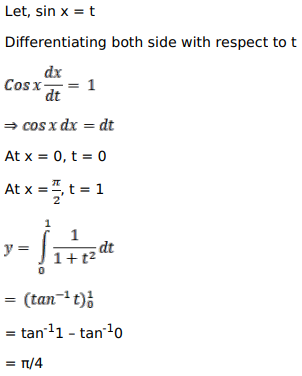# Mark against the correct answer in the following:

Question:

Mark $(\sqrt{)}$ against the correct answer in the following:

$\int_{0}^{\pi / 2} \frac{\cos x}{\left(1+\sin ^{2} x\right)} d x=?$

A. $\frac{\pi}{2}$

B. $\frac{\pi}{4}$

C. $\pi$

D. none of these

Solution: